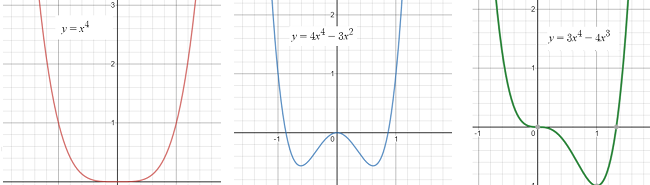# Quartic Regression

Share on

Quartic regression fits a quartic function (a polynomial function with degree 4) to a set of data. Quartic functions have the form:

f(x) = ax4 + bx3 + cx2 + dx + e.

For example:
f(x) = -.1072x4 + 13.2x3 – 380.1x2 – 154.2x + 998

The quartic function takes on a variety of shapes, with different inflection points (places where the function changes shape) and zero to many roots (places where the graph crosses the axis). For a > 0, three basic shapes are formed (graphed with Desmos.com):## Why Use Quartic Regression?

Quartic regression is another option for finding a line of best fit for data; It fits just as well as a cubic regression function and may even provide a better fit.

When modeling the data, the coefficient of determination (R2) will guide you when comparing different regression models (Aufmann & Nation, 2013). R-squared gives you the percentage variation in y explained by x-variables. The range is 0 to 1 (i.e. 0% to 100% of the variation in y can be explained by the x-variables). So when comparing models, the model with the higher R2 is the “better” model because it explains more variation in the model.

## How to Perform Quartic Regression on the TI 83

On the TI-83, follow the instructions for quadratic regression in the TI83 / TI89. The steps are exactly the same, except you choose #7 from the menu, instead of #5.

Note though, that you need at least five data points to fit a model to a quartic function.

## References

Aufmann, R. & Nation, R. Algebra & Trigonometry. Cengage Learning.
Hungerford, T. & Shaw, D. (2008). Contemporary Precalculus: A Graphing Approach. Cengage Learning.

CITE THIS AS:
Stephanie Glen. "Quartic Regression" From StatisticsHowTo.com: Elementary Statistics for the rest of us! https://www.statisticshowto.com/quartic-regression/
---------------------------------------------------------------------------Need help with a homework or test question? With Chegg Study, you can get step-by-step solutions to your questions from an expert in the field. Your first 30 minutes with a Chegg tutor is free!